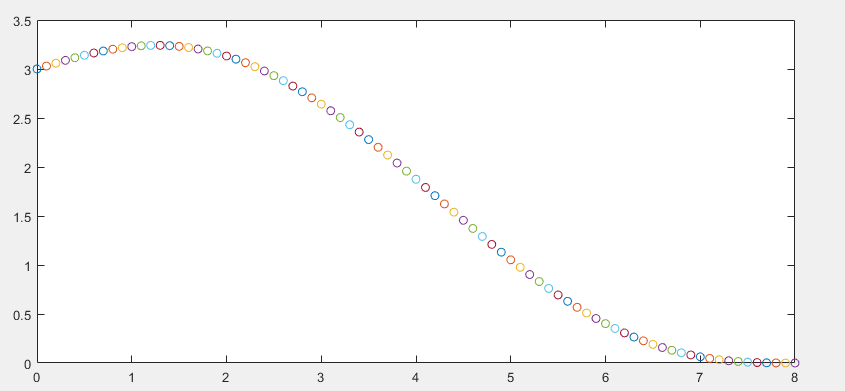# polynomial plotMatlab 同时被 2 个专栏收录30 篇文章 3 订阅

In this section, we provide a function that supports generate a quintic polynomial.

xs = 3;
vxs = 0.3;
axs = 0;
xe = 0;
vxe = 0;
axe = 0;
T = 8;
[a0, a1, a2, a3, a4,a5] = quintic_polynomial(xs, vxs, axs, xe, vxe, axe,T);

figure
for t = 0:0.1:T
d = a0 + a1*t + a2*t^2 + a3*t^3 + a4*t^4 + a5*t^5;
plot(t,d,'-o');
hold on
end

function [a0, a1, a2, a3, a4,a5] = quintic_polynomial(xs, vxs, axs, xe, vxe, axe,T)
A = [0,0,0,0,0,1; T^5,T^4,T^3,T^2,T,1;...
0,0,0,0,1,0 ; 5*T^4  4*T^3 3*T^2 2*T 1 0 ; ...
0 0 0 2 0 0; 20*T^3 12*T^2 6*T 2 0 0];
b = [xs, xe, vxs, vxe, axs, 0]';
x = A\b;
a5 = x(1);
a4 = x(2);
a3 = x(3);
a2 = x(4);
a1 = x(5);
a0 = x(6);
% A = [T^3 T^4 T^5; 3*T^2 4*T^3 5*T^4; 6*T 12*T^2 20*T^3];
% b = [(xe - xs  - vxs*T - 0.5*axs*T^2); (vxe- vxs - axs*T ); (axe - axs)];
% x = A\b;
% a0 = xs;
% a1 = vxs;
% a2 = axs/2;
%
% a3 = x(1);
% a4 = x(2);
% a5 = x(3);
end11-121万+
12-19568506-031万+
02-07563
06-244203
11-20
05-071万+
11-021万+
10-311万+
02-063万+
11-233052
04-02409
01-09531
04-202993
08-071300
07-269635
©️2020 CSDN 皮肤主题: Age of Ai 设计师:meimeiellie点击重新获取扫码支付1.余额是钱包充值的虚拟货币，按照1:1的比例进行支付金额的抵扣。
2.余额无法直接购买下载，可以购买VIP、C币套餐、付费专栏及课程。余额充值